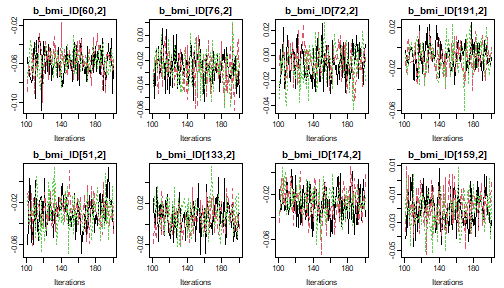# Parameter Selection

### How to specify which parameters to monitor and display in the results

#### 2020-06-22

In this vignette, we use the NHANES data for examples in cross-sectional data and the dataset simLong for examples in longitudinal data. For more info on these datasets, check out the vignette Visualizing Incomplete Data, in which the distribution of variables and missing values in both sets is explored.

Note:
In several of the examples we use n.adapt = 0 (and n.iter = 0, which is the default) in order to prevent the MCMC sampling to reduce computational some time. progress.bar = 'none' prevents printing of the progress of the MCMC sampling, which results in lengthy output in the vignette, but is useful in practice.

## Monitoring parameters

JointAI uses JAGS for performing the MCMC (Markov Chain Monte Carlo) sampling. Since JAGS only saves the values of MCMC chains for those parameters/variables for which the user has specified that they should be monitored, this is also the case in JointAI.

For this purpose, the main functions *_imp() have an argument monitor_params.

monitor_params takes a named list (often a named vector also works) with the following possible entries:

name/key word what is monitored
analysis_main betas and sigma_main, tau_main (in beta regression) or shape_main (in parametric survival models), D_main (in multi-level models) and basehaz (in proportional hazards models)
analysis_random ranef_main, D_main, invD_main, RinvD_main
other_models alphas, tau_other, gamma_other, delta_other
imps imputed values
betas regression coefficients of the main analysis model(s)
tau_main precision of the residuals from the analysis model(s)
sigma_main standard deviation of the residuals from the analysis model(s)
gamma_main intercepts in ordinal main model
delta_main increments of ordinal intercepts in main model(s)
ranef_main random effects of the analysis model(s)
D_main covariance matrix of the random effects of the main model(s)
invD_main inverse of D_main
RinvD_main scale matrix in Wishart prior for invD_main
alphas regression coefficients in the covariate models
tau_other precision parameters of the residuals from covariate models
gamma_other intercepts in ordinal covariate models
delta_other increments of ordinal intercepts in covariate models
ranef_other random effects of the covariate model(s)
D_other covariance matrix of the random effects of the covariate model(s)
invD_other inverse of D_other
RinvD_other scale matrix in Wishart prior for invD_other
other additional parameters

Each of the key words works as a switch, except for other, which should be a vector of character strings.

### Parameters of the analysis model

The default setting is monitor_params = c(analysis_main = TRUE), i.e., only the main parameters of the analysis model are monitored, and monitoring is switched off for all other parameters.

The main parameters are the regression coefficients of the analysis model (beta) and potentially the residual standard deviation, for example in linear or Gamma models, the second parameter in a beta distribution (parametrized with $$\mu$$ and $$\tau$$ instead of two shape parameters), the variance-covariance matrices of random effects used in the main models, the shape parameter in a parametric Weibull survival model, and the coefficients used in the spline specification of the baseline hazard of proportional hazards survival models.

The function parameters() returns the parameters that are specified to be followed (even for models where no MCMC sampling was performed, i.e. when n.iter = 0 and n.adapt = 0).

For example:

lm1 <- lm_imp(SBP ~ gender + WC + alc + creat, data = NHANES,  n.adapt = 0)

parameters(lm1)
#>   outcome outcat      varname      coef
#> 1     SBP   <NA>  (Intercept)   beta
#> 2     SBP   <NA> genderfemale   beta
#> 3     SBP   <NA>           WC   beta
#> 4     SBP   <NA>       alc>=1   beta
#> 5     SBP   <NA>        creat   beta
#> 6     SBP   <NA>         <NA> sigma_SBP

The column outcome refers to the response variable of a particular model, to distinguish between the regression coefficients in different sub-models, and the column outcat will contain the category of the response variable that a particular linear predictor corresponds to in multinomial logit models.

### Imputed values & parameters of the imputation models

To generate (multiple) imputed datasets that can be used for further analyses, the imputed values need to be monitored. This can be done by setting monitor_params = c(imps = TRUE).

lm2 <- lm_imp(SBP ~ age + WC + alc + smoke + occup,
data = NHANES, n.adapt = 0,
monitor_params = c(imps = TRUE, analysis_main = FALSE)
)

parameters(lm2)
#>    outcat varname            coef outcome
#> 1      NA      NA   M_lvlone[1,2]      NA
#> 2      NA      NA   M_lvlone[7,2]      NA
#> 3      NA      NA   M_lvlone[8,2]      NA
#> 4      NA      NA  M_lvlone[12,2]      NA
#> 5      NA      NA  M_lvlone[13,2]      NA
#> 6      NA      NA  M_lvlone[21,2]      NA
#> 7      NA      NA  M_lvlone[22,2]      NA
#> 8      NA      NA  M_lvlone[31,2]      NA
#> 9      NA      NA  M_lvlone[33,2]      NA
#> 10     NA      NA  M_lvlone[34,2]      NA
#> 11     NA      NA  M_lvlone[39,2]      NA
#> 12     NA      NA  M_lvlone[49,2]      NA
#> 13     NA      NA  M_lvlone[66,2]      NA
#> 14     NA      NA  M_lvlone[67,2]      NA
#> 15     NA      NA  M_lvlone[80,2]      NA
#> 16     NA      NA  M_lvlone[86,2]      NA
#> 17     NA      NA  M_lvlone[91,2]      NA
#> 18     NA      NA  M_lvlone[92,2]      NA
#> 19     NA      NA M_lvlone[105,2]      NA
#> 20     NA      NA M_lvlone[111,2]      NA
#> 21     NA      NA M_lvlone[115,2]      NA
#> 22     NA      NA M_lvlone[118,2]      NA
#> 23     NA      NA M_lvlone[120,2]      NA
#> 24     NA      NA M_lvlone[127,2]      NA
#> 25     NA      NA M_lvlone[132,2]      NA
#> 26     NA      NA M_lvlone[139,2]      NA
#> 27     NA      NA M_lvlone[146,2]      NA
#> 28     NA      NA M_lvlone[149,2]      NA
#> 29     NA      NA M_lvlone[152,2]      NA
#> 30     NA      NA M_lvlone[163,2]      NA
#> 31     NA      NA M_lvlone[165,2]      NA
#> 32     NA      NA M_lvlone[169,2]      NA
#> 33     NA      NA M_lvlone[180,2]      NA
#> 34     NA      NA M_lvlone[185,2]      NA
#> 35     NA      NA   M_lvlone[6,3]      NA
#> 36     NA      NA  M_lvlone[16,3]      NA
#> 37     NA      NA  M_lvlone[24,3]      NA
#> 38     NA      NA  M_lvlone[27,3]      NA
#> 39     NA      NA  M_lvlone[45,3]      NA
#> 40     NA      NA  M_lvlone[57,3]      NA
#> 41     NA      NA  M_lvlone[58,3]      NA
#> 42     NA      NA  M_lvlone[60,3]      NA
#> 43     NA      NA  M_lvlone[61,3]      NA
#> 44     NA      NA  M_lvlone[65,3]      NA
#> 45     NA      NA  M_lvlone[66,3]      NA
#> 46     NA      NA  M_lvlone[70,3]      NA
#> 47     NA      NA  M_lvlone[80,3]      NA
#> 48     NA      NA  M_lvlone[81,3]      NA
#> 49     NA      NA  M_lvlone[85,3]      NA
#> 50     NA      NA  M_lvlone[88,3]      NA
#> 51     NA      NA  M_lvlone[89,3]      NA
#> 52     NA      NA  M_lvlone[90,3]      NA
#> 53     NA      NA M_lvlone[101,3]      NA
#> 54     NA      NA M_lvlone[104,3]      NA
#> 55     NA      NA M_lvlone[116,3]      NA
#> 56     NA      NA M_lvlone[133,3]      NA
#> 57     NA      NA M_lvlone[137,3]      NA
#> 58     NA      NA M_lvlone[143,3]      NA
#> 59     NA      NA M_lvlone[168,3]      NA
#> 60     NA      NA M_lvlone[170,3]      NA
#> 61     NA      NA M_lvlone[180,3]      NA
#> 62     NA      NA M_lvlone[186,3]      NA
#> 63     NA      NA  M_lvlone[16,4]      NA
#> 64     NA      NA  M_lvlone[99,4]      NA
#> 65     NA      NA M_lvlone[123,4]      NA
#> 66     NA      NA M_lvlone[156,4]      NA
#> 67     NA      NA M_lvlone[158,4]      NA
#> 68     NA      NA M_lvlone[166,4]      NA
#> 69     NA      NA M_lvlone[172,4]      NA
#> 70     NA      NA  M_lvlone[33,5]      NA
#> 71     NA      NA M_lvlone[150,5]      NA

JointAI uses separate data matrices per level in the hierarchy of a multi-level model. The lowest level (and only level for models with no hierarchical structure) is called lvlone, the other levels are named based on the corresponding grouping/id variables.

For categorical variables the original variable as well as the corresponding dummy variables are stored in the matrices. The same is the case for interaction terms and transformations of variables.

head(lm2$data_list$M_lvlone)
#>          SBP alc occup smoke    WC (Intercept) age alc>=1 smokeformer smokecurrent
#> 10  108.0000  NA     1     1  99.0           1  35     NA          NA           NA
#> 14  105.3333   0     1     1  82.7           1  38     NA          NA           NA
#> 41  110.0000   0     3     1  94.9           1  78     NA          NA           NA
#> 77  106.0000   1     2     1  82.4           1  23     NA          NA           NA
#> 91  114.6667   0     3     1  93.1           1  40     NA          NA           NA
#> 105 139.3333   1    NA     3 105.4           1  54     NA          NA           NA
#>     occuplooking for work occupnot working
#> 10                     NA               NA
#> 14                     NA               NA
#> 41                     NA               NA
#> 77                     NA               NA
#> 91                     NA               NA
#> 105                    NA               NA

The parameters of the models for the incomplete variables can be selected with monitor_params = c(other_models = TRUE). This will set monitors for the regression coefficients (alpha) and other parameters, such as precision (tau_*) and intercepts & increments (gamma_* and delta_*) in cumulative logit models.

lm3 <- lm_imp(SBP ~ age + WC + alc + smoke + occup, data = NHANES, n.adapt = 0,
monitor_params = c(other_models = TRUE, analysis_main = FALSE)
)

parameters(lm3)
#>    outcome                outcat               varname           coef
#> 1      alc                  <NA>           (Intercept)       alpha
#> 2      alc                  <NA>                   age       alpha
#> 3      alc                  <NA>                    WC       alpha
#> 4      alc                  <NA>           smokeformer       alpha
#> 5      alc                  <NA>          smokecurrent       alpha
#> 6      alc                  <NA> occuplooking for work       alpha
#> 7      alc                  <NA>      occupnot working       alpha
#> 8    occup occuplooking for work           (Intercept)       alpha
#> 9    occup occuplooking for work                   age       alpha
#> 10   occup occuplooking for work                    WC      alpha
#> 11   occup occuplooking for work           smokeformer      alpha
#> 12   occup occuplooking for work          smokecurrent      alpha
#> 13   occup      occupnot working           (Intercept)      alpha
#> 14   occup      occupnot working                   age      alpha
#> 15   occup      occupnot working                    WC      alpha
#> 16   occup      occupnot working           smokeformer      alpha
#> 17   occup      occupnot working          smokecurrent      alpha
#> 18   smoke                  <NA>                   age      alpha
#> 19   smoke                  <NA>                    WC      alpha
#> 23   smoke                  <NA>                  <NA> gamma_smoke
#> 24   smoke                  <NA>                  <NA> gamma_smoke
#> 20      WC                  <NA>           (Intercept)      alpha
#> 21      WC                  <NA>                   age      alpha
#> 22      WC                  <NA>                  <NA>       sigma_WC

#### Side note: Getting information about of the imputation models

An overview of all models that form part of the joint model, including the names of the parameters and the hyper-parameters can be obtained with

list_models(lm2)
#> Linear model for "SBP"
#>    family: gaussian
#> * Predictor variables:
#>   (Intercept), age, WC, alc>=1, smokeformer, smokecurrent, occuplooking for
#>   work, occupnot working
#> * Regression coefficients:
#>   beta[1:8] (normal prior(s) with mean 0 and precision 1e-04)
#> * Precision of  "SBP" :
#>   tau_SBP (Gamma prior with shape parameter 0.01 and rate parameter 0.01)
#>
#>
#> Binomial model for "alc"
#>    family: binomial
#> * Reference category: "<1"
#> * Predictor variables:
#>   (Intercept), age, WC, smokeformer, smokecurrent, occuplooking for work,
#>   occupnot working
#> * Regression coefficients:
#>   alpha[1:7] (normal prior(s) with mean 0 and precision 1e-04)
#>
#>
#> Multinomial logit model for "occup"
#> * Reference category: "working"
#> * Predictor variables:
#>   (Intercept), age, WC, smokeformer, smokecurrent
#> * Regression coefficients:
#>   occuplooking for work: alpha[8:12]
#>   occupnot working: alpha[13:17] (normal prior(s) with mean 0 and precision 1e-04)
#>
#>
#> Cumulative logit model for "smoke"
#> * Reference category: "never"
#> * Predictor variables:
#>   age, WC
#> * Regression coefficients:
#>   alpha[18:19] (normal prior(s) with mean 0 and precision 1e-04)
#> * Intercepts:
#>   - never: gamma_smoke (normal prior with mean 0 and precision 1e-04)
#>   - former: gamma_smoke = gamma_smoke + exp(delta_smoke)
#> * Increments:
#>   delta_smoke (normal prior(s) with mean 0 and precision 1e-04)
#>
#>
#> Linear model for "WC"
#>    family: gaussian
#> * Predictor variables:
#>   (Intercept), age
#> * Regression coefficients:
#>   alpha[20:21] (normal prior(s) with mean 0 and precision 1e-04)
#> * Precision of  "WC" :
#>   tau_WC (Gamma prior with shape parameter 0.01 and rate parameter 0.01)

#### Side note: How to extract imputed datasets

Imputed datasets can be extracted and exported with the function get_MIdat(). A completed dataset is created by taking the imputed values from a randomly chosen iteration of the MCMC sample (transforming them back to the original scale, if scaling had been performed during the MCMC sampling) and filling them into the original, incomplete data.

get_MIdat() returns a long-format data.frame containing the imputed datasets (and possibly the original data) stacked onto each other. The imputation number is given in the variable Imputation_, column .id contains a newly created id variable for each observation in cross-sectional data (multi-level data should already contain an id variable).

get_MIdat() takes the arguments:

argument explanation
object ‘an object of class ’JointAI’
m number of datasets to be created
include logical; should the original data be included?
start the first iteration that may be randomly chosen (i.e., all previous iterations are discarded as burn-in)
minspace minimum number of iterations between iterations chosen as imputed values
seed optional seed value in order to make the random selection of iterations reproducible
export_to_SPSS logical; should the datasets be exported to SPSS, i.e., written as .txt and .sps file? If export_to_SPSS = FALSE (default) the imputed data is only returned data.frame
resdir directory the files are exported to
filename the name of the .txt and .sps files

### Random effects

For mixed models, analysis_main also includes the random effects covariance matrix D:

lme1 <- lme_imp(bmi ~ age + EDUC, random = ~age | ID, data = simLong, n.adapt = 0)

parameters(lme1)
#>   outcome outcat     varname          coef
#> 1     bmi   <NA> (Intercept)       beta
#> 2     bmi   <NA>     EDUCmid       beta
#> 3     bmi   <NA>     EDUClow       beta
#> 4     bmi   <NA>         age       beta
#> 5     bmi   <NA>        <NA>     sigma_bmi
#> 6     bmi   <NA>        <NA> D_bmi_ID[1,1]
#> 7     bmi   <NA>        <NA> D_bmi_ID[1,2]
#> 8     bmi   <NA>        <NA> D_bmi_ID[2,2]

Setting analysis_random = TRUE will switch on monitoring for the random effects (ranef), random effects covariance matrix (D), inverse of the random effects covariance matrix (invD) and the diagonal of the scale matrix of the Wishart-prior of invD (RinvD).

lme2 <- lme_imp(bmi ~ age + EDUC, random = ~age | ID, data = simLong, n.adapt = 0,
monitor_params = c(analysis_random = TRUE))

parameters(lme2)
#>    outcome outcat     varname              coef
#> 1      bmi   <NA> (Intercept)           beta
#> 2      bmi   <NA>     EDUCmid           beta
#> 3      bmi   <NA>     EDUClow           beta
#> 4      bmi   <NA>         age           beta
#> 5      bmi   <NA>        <NA>         sigma_bmi
#> 6      bmi   <NA>        <NA>          b_bmi_ID
#> 7      bmi   <NA>        <NA>  invD_bmi_ID[1,1]
#> 8      bmi   <NA>        <NA>  invD_bmi_ID[1,2]
#> 9      bmi   <NA>        <NA>  invD_bmi_ID[2,2]
#> 10     bmi   <NA>        <NA>     D_bmi_ID[1,1]
#> 11     bmi   <NA>        <NA>     D_bmi_ID[1,2]
#> 12     bmi   <NA>        <NA>     D_bmi_ID[2,2]
#> 13     bmi   <NA>        <NA> RinvD_bmi_ID[1,1]
#> 14     bmi   <NA>        <NA> RinvD_bmi_ID[2,2]

It is possible to select only a subset of the random effects parameters by specifying them directly, e.g.

lme3a <- lme_imp(bmi ~ age + EDUC, random = ~age | ID, data = simLong, n.adapt = 0,
monitor_params = c(analysis_main = TRUE, RinvD_main = TRUE))

parameters(lme3a)
#>    outcome outcat     varname              coef
#> 1      bmi   <NA> (Intercept)           beta
#> 2      bmi   <NA>     EDUCmid           beta
#> 3      bmi   <NA>     EDUClow           beta
#> 4      bmi   <NA>         age           beta
#> 5      bmi   <NA>        <NA>         sigma_bmi
#> 6      bmi   <NA>        <NA>     D_bmi_ID[1,1]
#> 7      bmi   <NA>        <NA>     D_bmi_ID[1,2]
#> 8      bmi   <NA>        <NA>     D_bmi_ID[2,2]
#> 9      bmi   <NA>        <NA> RinvD_bmi_ID[1,1]
#> 10     bmi   <NA>        <NA> RinvD_bmi_ID[2,2]

or by switching unwanted parts of analysis_random off, e.g.

lme3b <- lme_imp(bmi ~ age + EDUC, random = ~age | ID, data = simLong, n.adapt = 0,
monitor_params = c(analysis_main = TRUE,
analysis_random = TRUE,
RinvD_main = FALSE,
ranef_main = FALSE))
#> Warning:
#> It is currently not possible to use "contr.poly" for incomplete categorical
#> covariates. I will use "contr.treatment" instead.  You can specify (globally)
#> which types of contrasts are used by changing "options('contrasts')".

parameters(lme3b)
#>    outcome outcat     varname             coef
#> 1      bmi   <NA> (Intercept)          beta
#> 2      bmi   <NA>     EDUCmid          beta
#> 3      bmi   <NA>     EDUClow          beta
#> 4      bmi   <NA>         age          beta
#> 5      bmi   <NA>        <NA>        sigma_bmi
#> 6      bmi   <NA>        <NA> invD_bmi_ID[1,1]
#> 7      bmi   <NA>        <NA> invD_bmi_ID[1,2]
#> 8      bmi   <NA>        <NA> invD_bmi_ID[2,2]
#> 9      bmi   <NA>        <NA>    D_bmi_ID[1,1]
#> 10     bmi   <NA>        <NA>    D_bmi_ID[1,2]
#> 11     bmi   <NA>        <NA>    D_bmi_ID[2,2]

### Other parameters

The element other in monitor_params allows to specify one or multiple additional parameters to be monitored. When other is used with more than one element, monitor_params has to be a list.

Here, we monitor the probability to be in the alc>=1 group for subjects 1 through 3 and the expected value of the distribution of creat for the first subject. (This may not make a lot of sense here but being able to monitor any node in a JAGS model can be quite useful when investigating convergence issues.)

lm4 <- lm_imp(SBP ~ gender + WC + alc + creat, data = NHANES,
monitor_params = list(analysis_main = FALSE,
other = c('p_alc[1:3]', "mu_creat")))

parameters(lm4)
#>   outcat varname        coef outcome
#> 1     NA      NA  p_alc[1:3]     alc
#> 2     NA      NA mu_creat   creat

## Subsets of Parameters for Plots, Summaries, …

The functions summary(), traceplot(), densplot(), GR_crit() and MC_error() all have an argument subset. This argument allows us to select a subset of parameters to be shown in the output. Especially when not only the parameters of the main analysis model are followed, but also, for example, imputed values, looking at a subset may be desirable. For more details about these functions, see also the vignette After Fitting.

subset follows the same logic as monitor_params described above.

By default, only the parameters of the main analysis model are displayed if they were monitored:

# Run a model monitoring analysis parameters and imputation parameters
lm5 <- lm_imp(SBP ~ gender + WC + alc + creat, data = NHANES, n.iter = 100,
progress.bar = 'none', monitor_params = c(other_models = TRUE))

# model summary
summary(lm5)
#>
#> Bayesian linear model fitted with JointAI
#>
#> Call:
#> lm_imp(formula = SBP ~ gender + WC + alc + creat, data = NHANES,
#>     n.iter = 100, monitor_params = c(other_models = TRUE), progress.bar = "none")
#>
#>
#> Posterior summary:
#>                Mean     SD   2.5%  97.5% tail-prob. GR-crit MCE/SD
#> (Intercept)  81.088 9.4594 62.852 97.814      0.000    1.02 0.0577
#> genderfemale  0.315 2.5308 -4.366  5.680      0.933    1.02 0.0577
#> WC            0.311 0.0721  0.173  0.455      0.000    1.00 0.0577
#> alc>=1        6.288 2.3944  1.946 10.938      0.000    1.07 0.0657
#> creat         7.239 8.1750 -7.610 25.142      0.387    1.00 0.0577
#>
#> Posterior summary of residual std. deviation:
#>           Mean    SD 2.5% 97.5% GR-crit MCE/SD
#> sigma_SBP 14.4 0.789 12.9  15.9       1 0.0577
#>
#>
#> MCMC settings:
#> Iterations = 101:200
#> Sample size per chain = 100
#> Thinning interval = 1
#> Number of chains = 3
#>
#> Number of observations: 186

# traceplot of the MCMC sample
traceplot(lm5)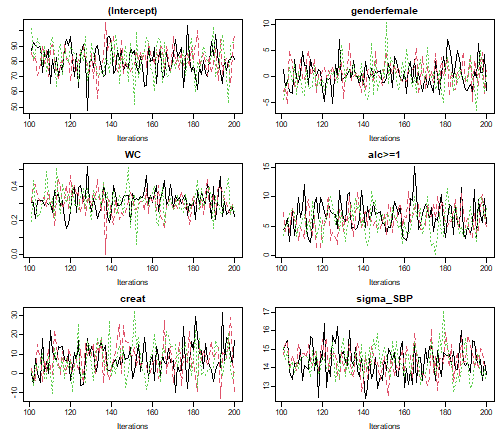# density plot of the MCMC sample
densplot(lm5)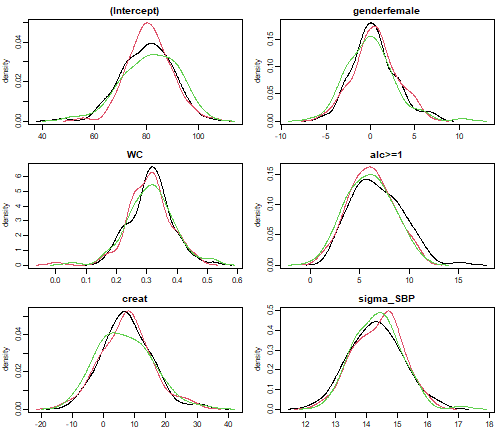# Gelman-Rubin criterion
GR_crit(lm5)
#> Potential scale reduction factors:
#>
#>              Point est. Upper C.I.
#> (Intercept)       1.008       1.02
#> genderfemale      1.005       1.02
#> WC                1.000       1.00
#> alc>=1            1.018       1.07
#> creat             0.999       1.00
#> sigma_SBP         0.999       1.00
#>
#> Multivariate psrf
#>
#> 1.03

# Monte Carlo Error of the MCMC sample
MC_error(lm5)
#>                est   MCSE    SD MCSE/SD
#> (Intercept)  81.09 0.5461 9.459   0.058
#> genderfemale  0.32 0.1461 2.531   0.058
#> WC            0.31 0.0042 0.072   0.058
#> alc>=1        6.29 0.1573 2.394   0.066
#> creat         7.24 0.4720 8.175   0.058
#> sigma_SBP    14.35 0.0456 0.789   0.058

When analysis_main was not switched on the default behaviour is that all parameters are displayed:

# Re-run the model from above, now creating MCMC samples
lm4 <- lm_imp(SBP ~ gender + WC + alc + creat,
data = NHANES, n.iter = 100, progress.bar = 'none',
monitor_params = list(analysis_main = FALSE,
other = c('mu_alc[1:3]', "mu_creat")))

traceplot(lm4, ncol = 4)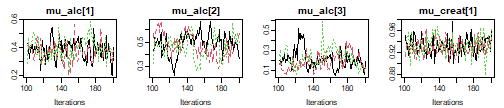### Select a subset of the variables to display

To display other parts of the MCMC sample, subset needs to be specified:

# we use lm5 from above
GR_crit(lm5, subset = c(analysis_main = FALSE, other_models = TRUE))
#> Potential scale reduction factors:
#>
#>                     Point est. Upper C.I.
#> alc: (Intercept)          1.02       1.06
#> alc: genderfemale         1.03       1.07
#> alc: WC                   1.02       1.05
#> alc: creat                1.03       1.07
#> creat: (Intercept)        1.00       1.00
#> creat: genderfemale       1.00       1.01
#> creat: WC                 1.00       1.01
#> WC: (Intercept)           1.00       1.00
#> WC: genderfemale          1.01       1.01
#> sigma_creat               1.00       1.02
#> sigma_WC                  1.01       1.03
#>
#> Multivariate psrf
#>
#> 1.06

To select only some of the parameters, they can be specified directly by name via the other element of subset:

summary(lm5, subset = list(other = c('creat', 'alc>=1')))
#>
#> Bayesian linear model fitted with JointAI
#>
#> Call:
#> lm_imp(formula = SBP ~ gender + WC + alc + creat, data = NHANES,
#>     n.iter = 100, monitor_params = c(other_models = TRUE), progress.bar = "none")
#>
#>
#> Posterior summary:
#>                Mean     SD   2.5%  97.5% tail-prob. GR-crit MCE/SD
#> (Intercept)  81.088 9.4594 62.852 97.814      0.000    1.02 0.0577
#> genderfemale  0.315 2.5308 -4.366  5.680      0.933    1.02 0.0577
#> WC            0.311 0.0721  0.173  0.455      0.000    1.00 0.0577
#> alc>=1        6.288 2.3944  1.946 10.938      0.000    1.07 0.0657
#> creat         7.239 8.1750 -7.610 25.142      0.387    1.00 0.0577
#>
#> Posterior summary of residual std. deviation:
#>           Mean    SD 2.5% 97.5% GR-crit MCE/SD
#> sigma_SBP 14.4 0.789 12.9  15.9       1 0.0577
#>
#>
#> MCMC settings:
#> Iterations = 101:200
#> Sample size per chain = 100
#> Thinning interval = 1
#> Number of chains = 3
#>
#> Number of observations: 186

Note that the model summary will contain separate parts per sub-model when regression coefficients from different models are monitored.

This also works when a subset of the imputed values should be displayed:

# Re-run the model from above, now creating MCMC samples
lm2 <- lm_imp(SBP ~ age + WC + alc + smoke + occup,
data = NHANES, n.iter = 100, progress.bar = 'none',
monitor_params = c(imps = TRUE, analysis_main = FALSE)
)

# select only imputed values for 'WC' (4th column of Wc)
sub3 <- grep('M_lvlone\$[[:digit:]]+,5\$', parameters(lm2)$coef, value = TRUE) sub3 #>  "M_lvlone[33,5]" "M_lvlone[150,5]" traceplot(lm2, subset = list(other = sub3), ncol = 2)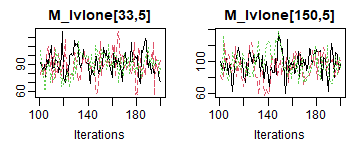### Random subset of subject-specific values When the number of imputed values is larger, or in order to check convergence of random effects, it may not be feasible to plot all trace plots. In that case, a random subset of, for instance, the random effects can be selected: lme4 <- lme_imp(bmi ~ age + EDUC, random = ~age | ID, data = simLong, n.iter = 100, progress.bar = 'none', monitor_params = c(analysis_main = FALSE, ranef_main = TRUE)) # exract random intercepts ri <- grep('^b_bmi_ID\$[[:digit:]]+,1\$$', colnames(lme4$MCMC[]), value = T) # extract random slopes rs <- grep('^b_bmi_ID\$[[:digit:]]+,2\$$', colnames(lme4\$MCMC[]), value = T)

# plot the chains of 8 randomly selected random intercepts
traceplot(lme4, subset = list(other = sample(ri, size = 8)), ncol = 4)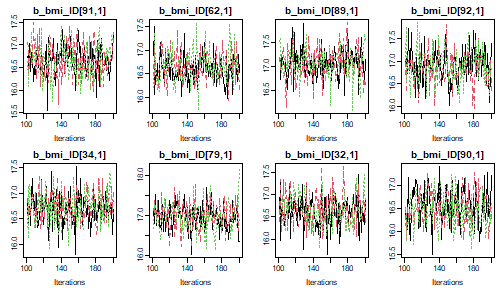# plot the chains of 8 randomly selected random slopes
traceplot(lme4, subset = list(other = sample(rs, size = 8)), ncol = 4)